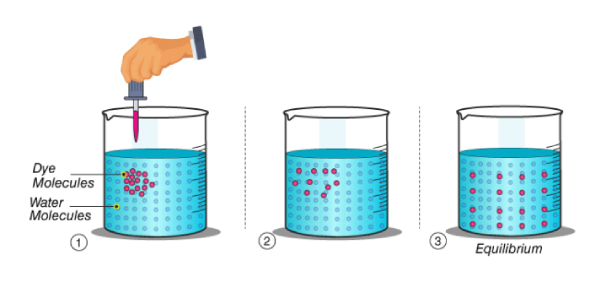# Characteristics Of Matter Quiz: Chemistry Trivia!

10 Questions | Total Attempts: 1367SettingsThis Basic Chemistry quiz has been designed for students to test their knowledge and understanding of the concepts involved with the matter, including states of matter, changes in matter, mass, volume, density, etc. There is a list of 10 questions. Read the questions carefully and answer.

• 1.
Which is an example of a physical change?
• A.

Burning paper

• B.

Melting an ice cube

• C.

A car that is rusting

• D.

Mixing baking soda with vinegar

• 2.
What happens when water freezes?
• A.

A liquid becomes a gas

• B.

A gas becomes a liquid

• C.

A liquid becomes a solid

• D.

A solid becomes a liquid

• 3.
Which is an example of a chemical change?
• A.

Tearing paper

• B.

Melting glass

• C.

Mixing chloring gas and sodium to make salt

• D.

• 4.
The matter has three states. Which of the following is not one of those states?
• A.

Density

• B.

Solid

• C.

Gas

• D.

Liquid

• 5.
Which of the following properties can only be determined through a chemical change?
• A.

Explosiveness

• B.

Melting point

• C.

Colour

• D.

Mass

• 6.
The matter is defined as anything that:
• A.

Has a fixed volume and weight

• B.

Has energy

• C.

Has mass and takes up space

• D.

Can be weighed on a balance

• 7.
Which of the following is true for all chemical reactions?
• A.

The total mass of the products decreases

• B.

The total mass of the reactants equals the total mass of the products

• C.

The total mass of the reactants increases

• D.

The total mass of the products is greater than the total mass of the reactants

• 8.
Which of the following is NOT an example of matter?
• A.

Smoke

• B.

Heat

• C.

Water

• D.

Water vapour

• 9.
All of the following changes to a substance are chemical changes EXCEPT:
• A.

Reacting

• B.

Tarnishing

• C.

Burning

• D.

Boiling

• 10.
Which of the following is a valid difference between a mixture and a compound?
• A.

A compound is made up of more than one phase

• B.

A mixture can only be separated into its components by chemical means

• C.

A mixture must be uniform in composition

• D.

A compound can only be separated into its components by chemical means.

Related TopicsBack to top